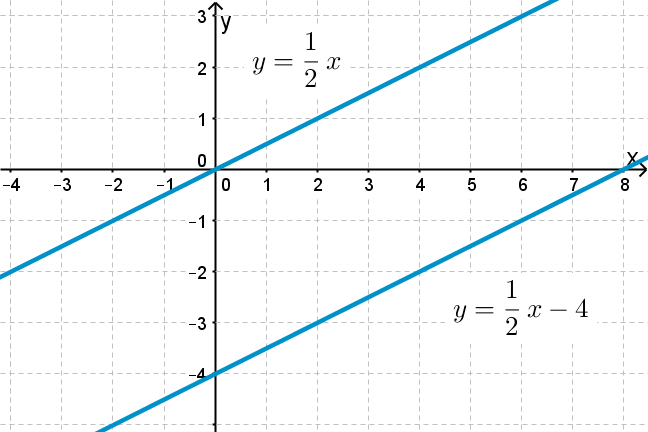1.13

## UNSW Sydney# Transformations of lines

We now apply translations and dilations to lines, or more precisely to the equations of those lines. What happens to the equation of a line if we translate it by $\normalsize{2}$ in the $\normalsize{x}$ direction, or by $\normalsize{-3}$ in the $\normalsize{y}$ direction? What happens if we apply a dilation $\normalsize{[x,y]}$ goes to $\normalsize{[rx,ry]}$?

In this step you will learn

• how a translation in either the $\normalsize{x}$ or $\normalsize{y}$ direction affects the equation of a line

• how a dilation affects the equation of a line.

## Translating a line

If we take the line $\normalsize{y=3x-1}$ and translate it up by $\normalsize{2}$ in the $\normalsize{y}$-direction, we get $\normalsize{y=3x-1+2}$ or just

If we take the same line $\normalsize{y=3x-1}$ and translate it by $\normalsize{2}$ in the $\normalsize{x}$ direction, then the equation changes more subtly to $\normalsize{y=3(x-2)-1}$. Can you see why? Suppose the point $\normalsize{[r,s]}$ lies on the original line. This means that

But then $\normalsize{[r+2,s]}$ will lie on the translated line ${\normalsize y = 3(x-2)-1 = 3x-7}$.

Q1 (E): Which of the following equations represents a translation of $\normalsize{y=3x-1}$ by $\normalsize{3}$ in the negative $\normalsize{x}$ direction?

a) $\normalsize{y=3(x-3)-1}$
b) $\normalsize{y=3(x+3)-1}$

Q2 (M): Find the equation of the red line, and hence find the equation of its translate the blue line.Summarizing, we have the following:

Replacing $\normalsize{y}$ by $\normalsize{y-k}$ in an equation represents a translation by $\normalsize{k}$ in the $\normalsize{y}$ direction.

Replacing $\normalsize{x}$ by $\normalsize{x-h}$ in an equation represents a translation by $\normalsize{h}$ in the $\normalsize{x}$ direction.

## Scaling a line

If we multiply the $\normalsize{y}$ coordinate of a point $\normalsize{[x,y]}$ which lies on the line $\normalsize{y=3x-1}$ (in red) by $\normalsize{\frac{3}{2}}$, the effect is a dilation in the $\normalsize{y}$ direction by just this factor. This takes the line $\normalsize{y=3x-1}$ to $\normalsize{y=\frac{9}{2}x-\frac{3}{2}}$, which is the blue line below.If we multiply the $\normalsize{x}$ coordinate of a point $\normalsize{[x,y]}$ on the line $\normalsize{y=3x-1}$ by $\normalsize{\frac{1}{2}}$, the effect is a dilation in the $\normalsize{x}$ direction by just this factor.Summarizing, we have the following:

Replacing $\normalsize{y}$ by $\normalsize{ry}$ in an equation represents a dilation by $\normalsize{\frac{1}{r}}$ in the $\normalsize{y}$ direction.

Replacing $\normalsize{x}$ by $\normalsize{sx}$ in an equation represents a dilation by $\normalsize{\frac{1}{s}}$ in the $\normalsize{x}$ direction.

## Combining translation and dilation

So what happens if we combine a translation by $\normalsize{3}$ in the $\normalsize{y}$ direction with a dilation by $\normalsize{4}$ in the $\normalsize{x}$ direction? The line $\normalsize{y=3x-1}$ first goes to $\normalsize{(y-3)=3x-1}$ and then to $\normalsize{(y-3)=3(x/4)-1}$. This works out to be $\normalsize{y=(3/4)x+2}$.A1. The translate by $\normalsize{-3}$ in the $\normalsize{x}$ direction is b) $\normalsize{y=3(x+3)-1}$. This simplifies to the equation $\normalsize{y=3x+8}$.
A2. The point $[0,5]$ lies on the red line, and so its equation is of the form $y=kx+5$ for some number $k$. Since the point $[-3,-4]$ also lies on this line, this becomes $y=3x+5$. The blue line is the translate in the $x$ direction of the red line by $\normalsize{4}$, and therefore has equation $y=3(x-4)+5$ or $y=3x-7$.